# Brick weight

The brick weighs 2 kg and a half bricks.

How much does one brick weigh?

x =  4 kg

### Step-by-step explanation: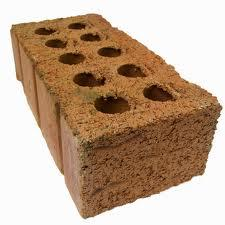Did you find an error or inaccuracy? Feel free to write us. Thank you!Math student
half bricks or half brick?
Or may be better, the weight of a half brick.Dr Math
we updated spelling of this math problem, thank you!Math student
needs rephrasingTips to related online calculators
Do you have a linear equation or system of equations and looking for its solution? Or do you have a quadratic equation?

## Related math problems and questions:

• Bricks II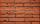3/4 bricks weighs 6 kg and 2/3 bricks. How weighs one whole brick?
• Two bricks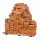One brick weighs 1kg and half a brick, how many weigh two bricks?
• Can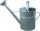Watering can full of water weighs 10 kg. Half-full can weighs 5.5 kg. How much weigh can?
• Brick weightHalf the weight of a brick plus 20 pounds is equal to 1/3 the weight of the brick plus 30 pounds. How much does the brick weigh?
• Brick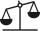Isosceles scale has on one side all brick and second weight 1 kg and 1/4 of brick. The balance is in equilibrium. What is the weight of a brick?
• Brick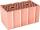Brick weight before drying is 5.3 kg. After drying, the bricks is 1 kg lighter and after firing is 0.7 kg lighter than after drying. What is the weight of the brick after drying and firing?
• Bag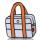Nelly found interesting point. An empty bag weighs 4 kg less than full. And empty bag is 5 times easier than full. How many kg weigh things in this bag?
• BricksOpenings in perforated bricks occupy 10% and brick has dimensions 30 cm, 15 cm and 7.5 cm. Calculate a) the weight of a perforated bricks, if you know that the density of the full brick material is p = 1800 kg/m3 (1.8 kg/dm3) b) the number of perforated
• Bob and Bobek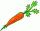Bobek has 2 cabbages and 5 carrots, Bob has 2 cabbages and 9 carrots. When Bobek stepped on the scale weight 5800 g and when Bob weight 8960 g. Actual Bob weight is 8 kg and Bobek 5 kg. How much weighs one carrot?
• Children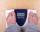Margaret and Zdena weigh the same and Petra 3 kg more. Together weigh 156 kg. How much do they weigh?
• Milk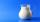Mum bought 16 cartons of milk. One carton of milk weighs 0.925 kg. How many weight all purchase?
• One kilogramThe apple weighs 125 grams and half apple. How many apples weigh 1 kilogram?
• Freight train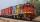Twenty-five identical freight wagons are connected behind the locomotive. The weight of the locomotive and all wagons is 1009000 kg. The locomotive weighs 84 tons. How many kilograms does one freight car weigh?
• Buns and toastsTwo buns weigh 10 grams more than two toasts. One Bun and two Toast weigh a total of 110 grams. How many grams weigh three toasts? How many grams does a Bun weigh?
• Wood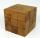Wood cube with edge 11 cm weights 0.753 kg. What weight have 10, 100 and 1000 these cubes?
• BucketThe bucket half filled with water weighs 5.55 kg, the full bucket weighs 9.85 kg. How much does the bucket weigh?
• HomeworkMother and Kate together weigh 24.5 kg more than the father. Father weighs 7.6 kg more than mother. Father weight 44.8 kg more than Kate. Determine weights of father mother and Kate.